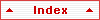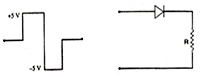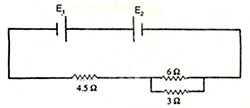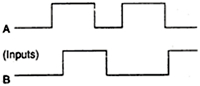# PhysicsNumerical Questions (2 marks) The output of an OR gate is connected to both the inputs of a NAND gate. Draw the logic circuit of this combination of gates and write its truth table. A convex lens of refractive index 1.5 has a focal length of 18 cm in air. Calculate the change in its focal length when it is immersed in water of refractive index 1.33. Two point charges and are separated by a distance of 1 m in air. Calculate at what point on the line joining the two charges is the electric potential zero. A voltage of 30 V is applied across a carbon resistor with first, second and third rings of blue, black and yellow colours respectively. Calculate the value of current, in mA, through the resistor. Calculate the current drawn by the primary of a transformer which steps down 200 V to 20 V to operate a device of resistance. Assume the efficiency of the transformer to be 80%. An a.c. voltage of 100 V, 50 Hz is connected across a 20 ohm resistor and mH inductor in series. Calculate impedance of the circuit, rms current in the circuit In an ammeter (consisting of a galvanometer and a shunt), 0.5% of the main current passes through the galvanometer. Resistance of the galvanometer coil is G. Calculate the resistance of the shunt in terms of galvanometer resistance, G. An electric bulb B and a parallel plate capacitor C are connected in series to the a.c. mains as shown in the given figure. The bulb glows with some brightness. How will the glow of the bulb be affected on introducing a dielectric slab between the plates of the capacitor? Give reasons in support of your answer. The output of a 2-input NOR gate is fed to a NOT gate. Draw the logic circuit of this combination of gates and write the truth table for the output of the combination for all inputs. The electric field and electric potential at any point due to a point charge kept in air is 20 N/C and 10 J/C respectively. Compute the magnitude of this charge. Draw and explain the output waveform across the load resistor R, if the input waveform is as shown in the given figure.Two cells E1 and E2 in the given circuit diagram have an emf of 5 V and 9 V and internal resistance of 0.3Ω and 1.2Ω respectively.Calculate the value of current flowing through the resistance of 3Ω. Two capacitors of capacitance of 6µF and 12µF are connected in series with a battery. The voltage across the 6µF capacitor is 2 V. Compute the total battery voltage. A parallel plate capacitor with air between the plates has a capacitance of 8 pF. The separation between the pates is now reduced by half and the space between them is filled with a medium of dielectric constant 5. Calculate the value of capacitance of the capacitor in the second case. A 4µF capacitor is charged by a 200 V supply. The supply is then disconnected and the charged capacitor is connected to another uncharged 2 µF capacitor. How much electrostatic energy of the first capacitor is lost in the process of attaining the steady situation? Find the wavelength of electromagnetic waves of frequency 5 x 1019Hz in free space. A compound microscope with an objective of 1.0 cm focal length and an eye-piece of 2.0 cm focal length has a tube length of 20 cm. Calculate the magnifying power of the microscope, if the final image is formed at the near point of the eye. The magnifying power of an astronomical telescope in the normal adjustment position is 100. The distance between the objective and the eye-piece is 101 cm. Calculate the focal lengths of the objective and of the eye-piece. An electric dipole of length 4 cm, when placed with its axis making an angle of 600 with a uniform electric field experiences a torque 4√3 Nm. Calculate the magnitude of the electric field. potential energy of the dipole, if the dipole has charges of ±8nC. ATV tower has a height of 400 m at a given place. Calculate coverage range, if the radius of the earth is 6400 km. Give the logic symbol for an OR gate. Draw the output wave form for input wave forms A and B for this gate.In the figure below, circuit symbol of a logic gate and input wave from is shown. Name the logic gate, write its truth table and give the output wave form. Two point charges qA= +3µc and qB= -3µc are located 20 cm apart in vacuum, Find the electric field at the mid -point of the line AB joining the two charges, If a negative test charge of magnitude1.5×10-9Cis placed at the centre, find the force experienced by the test charge.

Due to time constraint we cannot solve the entire question paper. But, we can help you in the questions which you think are really difficult. Please mention the same and go through our study material and revision notes to solve the remaining ones on your own.

• -22
What are you looking for?